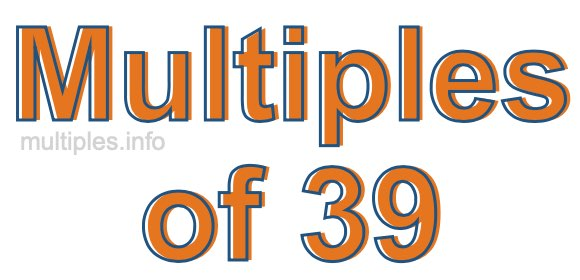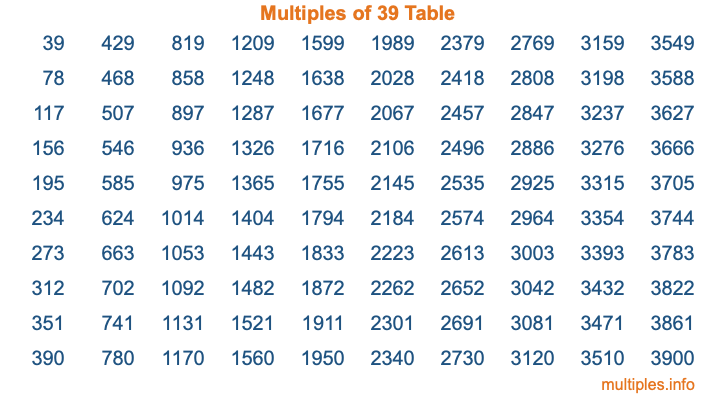Multiples of 39Welcome to the Multiples of 39 page. Here we will first teach you everything you will ever need to know about the multiples of 39, and then give you a study guide summary of everything we taught you to make sure you remember it all. Use this page to look up facts and learn information about the multiples of 39. This page will make you a multiples of thirty-nine expert!

Definition of Multiples of 39
Multiples of 39 are all the numbers that when divided by 39 equal an integer. Each of the multiples of 39 are called a multiple. A multiple of 39 is created by multiplying 39 by an integer.

Therefore, to create a list of multiples of 39, you start with 1 multiplied by 39, then 2 multiplied by 39, then 3 multiplied by 39, and so on for as long as you want. Thus, the list of the first five multiples of 39 is 39, 78, 117, 156, and 195. To see a larger list of multiples of 39, see the printable image of Multiples of 39 further down on this page. We also have a category where you can choose any nth multiple of 39.

Multiples of 39 Checker
The Multiples of 39 Checker below checks to see if any number of your choice is a multiple of 39. In other words, it checks to see if there is any number (integer) that when multiplied by 39 will equal your number. To do that, we divide your number by 39. If the the quotient is an integer, then your number is a multiple of 39.

Is  a multiple of 39?

Least Common Multiple of 39 and ...
A Least Common Multiple (LCM) is the lowest multiple that two or more numbers have in common. This is also called the smallest common multiple or lowest common multiple and is useful to know when you are adding our subtracting fractions. Enter one or more numbers below (39 is already entered) to find the LCM.

Check out our LCM Calculator if you need more details about the Least Common Multiple or if you need the LCM for different numbers for adding and subtraction fractions.

nth Multiple of 39
As we stated above, 39 is the first multiple of 39, 78 is the second multiple of 39, 117 is the third multiple of 39, and so on. Enter a number below to find the nth multiple of 39.

th multiple of 39

Multiples of 39 vs Factors of 39
39 is a multiple of 39 and a factor of 39, but that is where the similarities end. All postive multiples of 39 are 39 or greater than 39. All positive factors of 39 are 39 or less than 39.

Below is the beginning list of multiples of 39 and the factors of 39 so you can compare:

Multiples of 39: 39, 78, 117, 156, 195, etc.

Factors of 39: 1, 3, 13, 39

As you can see, the multiples of 39 are all the numbers that you can divide by 39 to get a whole number. The factors of 39, on the other hand, are all the whole numbers that you can multiply by another whole number to get 39.

It's also interesting to note that if a number (x) is a factor of 39, then 39 will also be a multiple of that number (x).

Multiples of 39 vs Divisors of 39
The divisors of 39 are all the integers that 39 can be divided by evenly. Below is a list of the divisors of 39.

Divisors of 39: 1, 3, 13, 39

The interesting thing to note here is that if you take any multiple of 39 and divide it by a divisor of 39, you will see that the quotient is an integer.

Multiples of 39 Table
Below is an image of the first 100 multiples of 39 in a table. The table is in chronological order, column by column. The first column has the first ten multiples of 39, the second column has the next ten multiples of 39, and so on.The Multiples of 39 Table is also referred to as the 39 Times Table or Times Table of 39. You are welcome to print out our table for your studies.

Negative Multiples of 39
Although not often discussed or needed in math, it is worth mentioning that you can make a list of negative multiples of 39 by multiplying 39 by -1, then by -2, then by -3, and so on, to get the following list of negative multiples of 39:

-39, -78, -117, -156, -195, etc.

Multiples of 39 Summary
Below is a summary of important Multiples of 39 facts that we have discussed on this page. To retain the knowledge on this page, we recommend that you read through the summary and explain to yourself or a study partner why they hold true.

There are an infinite number of multiples of 39.

A multiple of 39 divided by 39 will equal a whole number.

39 divided by a factor of 39 equals a divisor of 39.

The nth multiple of 39 is n times 39.

The largest factor of 39 is equal to the first positive multiple of 39.

39 is a multiple of every factor of 39.

39 is a multiple of 39.

A multiple of 39 divided by a divisor of 39 equals an integer.

39 divided by a divisor of 39 equals a factor of 39.

Any integer times 39 will equal a multiple of 39.

Multiples of a Number
Here you can get the multiples of another number, all with the same attention to detail as we did for multiples of 39 on this page.

Multiples of
Multiples of 40
Did you find our page about multiples of thirty-nine educational? Do you want more knowledge? Check out the multiples of the next number on our list!